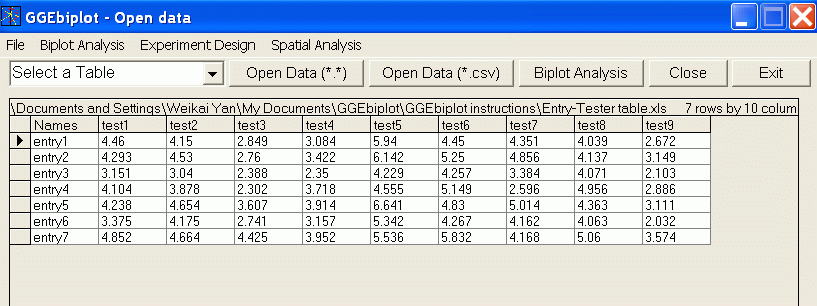Contents - Index

Main Menu MODELS - old

See Functions under MODELS-new for more options

For each two-way tables, biplots can be generated on any of the SIX built-in models:

• Model 0 generates biplots based on singular value decomposition of the grand-mean centered data.
• Model 1 generates biplots based on singular value decomposition of tester-centered data. It is used for dataset in which all testers have the same unit, such as a genotype-by-environment table of a single trait. This model may be called "GGE1".
• Model 2 generates biplots based on singular value decomposition of within-tester standard deviation standardized data. It is mostly used for datasets in which different units have different testers (such as a genotype-by-trait table). It can also be used in analyzing a two-way table of any trait when all testers are assumed to be equally important. This model may be called "GGE2".
• Model 3 generates biplots based on singular value decomposition of within-tester standard error standardized data. This option is available only when the data are replicated so that the standard error for each tester can be calculated. This model supposedly can remove any heterogeneity among the testers. This model may be called "GGE3".
• Model 4 generates biplots based on singular value decomposition of entry and tester double-centered data. This mode is effective in the following situations: 1) to study the genotype by environment interaction (GE) of a multi-environment dataset; 2) to study the specific combing ability (SCA) of a diallel cross dataset; and 3) to analyze gene expression data (or microarray data) to identify gene-treatment correspondences.
• Model 5 generates biplots based on the original two-way table. This model is essential for the so called covariate-effect biplot analysis.

Hint: For GGE biplot analysis, Model 3 is preferred and should be used as soon as feasible. See Yan et al. (2008).

Note: Biplots based on different models are compared through the above links using this dataset: